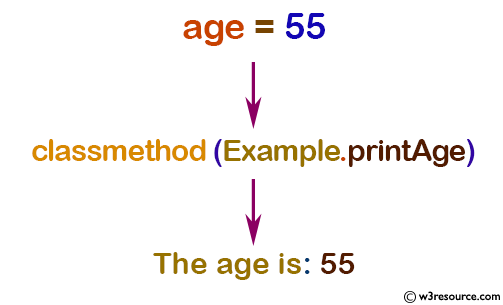# Python: classmethod() function

## classmethod() function

The classmethod() function is used to convert a method into a class method.

Syntax:

```classmethod(function)
```

A class method receives the class as implicit first argument, just like an instance method receives the instance. To declare a class method, use this idiom:

``````class C:
@classmethod
def f(cls, arg1, arg2, ...): ...
``````

The @classmethod form is a function decorator. It can be called either on the class (such as C.f()) or on an instance (such as C().f()). The instance is ignored except for its class. If a class method is called for a derived class, the derived class object is passed as the implied first argument.

Version:

(Python 3.2.5)

Parameter:

Name Description Required /
Optional
function Function that needs to be converted into a class method Required

Return value:

Return a class method for function.

Example: Create class method using classmethod()

``````class Example:
age = 55

def printAge(cls):
print('The age is:', cls.age)

# create printAge class method
Example.printAge = classmethod(Example.printAge)

Example.printAge()
``````

Output:

```The age is: 55
```

Pictorial Presentation:Python Code Editor:

Previous: chr()
Next: compile()

Test your Python skills with w3resource's quiz

﻿

## Python: Tips of the Day

How do I check if a list is empty?

For example, if passed the following:

```a = []
if not a:
print("List is empty")
```

Ref: https://bit.ly/2A4JXx9